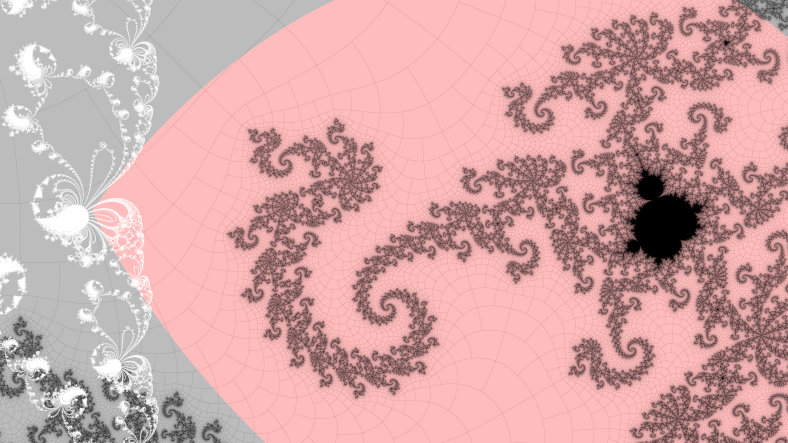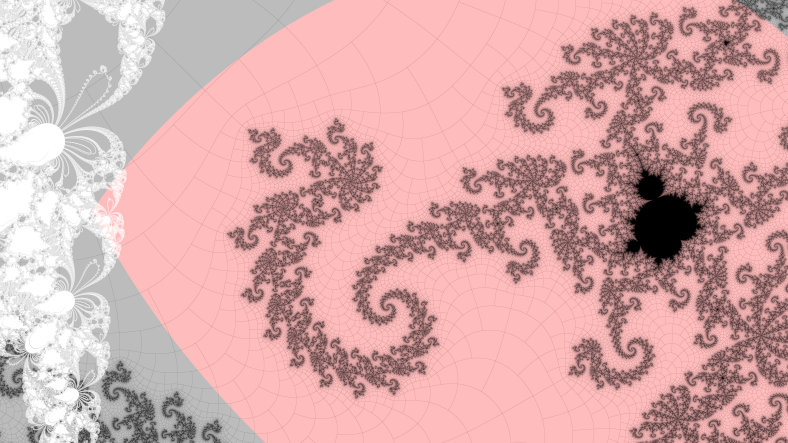# mathr / blog / #

## Atom domains and Newton basins

The Mandelbrot set $$M$$is formed by iterations of the function $$z \to z^2 + c$$ starting from $$z = 0$$. If this remains bounded for a given $$c$$, then $$c$$ is in $$M$$, otherwise (if it escapes to infinity) then $$c$$ is not in $$M$$. The interior of $$M$$ is characterized by collections of cardioid-like and disk-like shapes, these are hyperbolic components each associated with an integer, its period. For the $$c$$ at the center of each component, if the period is $$p$$ then after $$p$$ iterations, $$z$$ returns to $$0$$, and the iterations repeat (hence the name period). For points in the complex plane (either in $$M$$ or not) sufficiently near to a hyperbolic compoenent of period $$p$$, $$|z|$$ reaches a new minimum (discounting the initial $$z=0$$) at iteration $$p$$. The region for which this is true is called the atom domain associated with the hyperbolic component.

To find the center (sometimes called nucleus) of a hyperbolic component, one can use Newton's root-finding method in one complex variable. Iterate the derivative with respect to $$c$$ along with $$z$$ (using $$\frac{\partial z}{\partial c} \to 2 \frac{\partial z}{\partial c} z + 1$$) for $$p$$ iterations, then update $$c \to c - \frac{z}{\frac{\partial z}{\partial c}}$$ until it converges. However, Newton's method requires a good initial guess for $$c$$. As there are multiple roots, and if you are near to a root Newton's method brings you nearer to it, there must be a boundary where which root is reached depends sensitively on the initial guess. It turns out (if there are more than 2 roots) that the boundary is fractal, and for any point on the boundary, an arbitrarily small neighbourhood will be spread to all the roots. See 3blue1brown's videos on YouTube about the Newton fractal for further information. Which comes to my conjecture:

Conjecture: points in the complement of the Mandelbrot in an atom domain of period $$p$$ are good initial guesses for Newton's method to find the root of period $$p$$ at the center of that atom domain.

It turns out that this conjecture is false. The proof is by counter-example. The counter-example is the period $$18$$ island with angled internal address $$1 \to_{1/2} 2 \to_{1/8} 16 \to_{1/2} 18$$, whose upper external angle is $$.(010101010101100101)$$ when expressed in binary. I found this counter-example by brute force search: for every period increasing from $$1$$, trace every ray pair of that period until the endpoints reach the atom domain. Then from each use Newton's method to find the center of the hyperbolic component. Compare the two centers reached, if they aren't the same then we have found a counter-example. Here is a picture:The Mandelbrot set is shown in black, using distance estimation to make its filaments visible. The fractal boundary of the Newton basins of period $$18$$ is shown in white. The atom domain is shown in red. The complement of the Mandelbrot set is shown with a binary decomposition grid that follows the external rays and equipotentials. You can see that the path of the ray that goes from the cusp of the mini Mandelbrot island will intersect the Newton basin boundary at the corner of the atom domain, so that the eventual point of convergence of Newton's method is unpredictable. In my experiment it converged to the child bulb with angled internal address $$1 \to_{1/2} 2 \to_{1/9} 18$$.

The above image was using regular Newton's method, without factoring out the roots of lower period that divide the target period. With the reduced polynomials, the basins are typically a little bigger, but in this case it made no difference and the problem persists with this counter-example:I uploaded a short video showing the counter-example with both variants of Newton's method: watch on diode.zone. You can download the FragM source code for the visualisation.

This counter-example shows that the strategy of tracing rays until the atom domain is reached, before switching to Newton's method to find the root, is unsafe. A guaranteed safe strategy remains to be investigated.